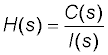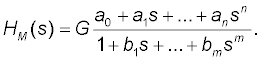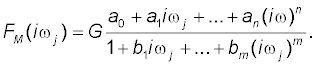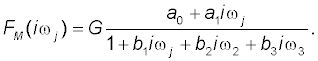GET THE APP

Mathematical Models of the Pharmacokinetic Behavior of Digoxin in Five Healthy Subjects following Rapid Intravenous Injection of 1 mg of Digoxin# Journal of Developing DrugsOpen Access

Research Article - (2015) Volume 4, Issue 5

# Mathematical Models of the Pharmacokinetic Behavior of Digoxin in Five Healthy Subjects following Rapid Intravenous Injection of 1 mg of Digoxin

Mária Durišová*
Department of Pharmacology of Inflammation, Institute of Experimental Pharmacology and Toxicology, Slovak Academy of Sciences, Slovak Republic
*Corresponding Author: Mária Durišová, Department of Pharmacology of Inflammation, Institute of Experimental Pharmacology and Toxicology, Slovak Academy of Sciences, Dúbravská Cesta 9, 84104 Bratislava, Slovak Republic, Tel: +421-2-54775928 Email:

## Abstract

Objectives: The main objective of the current study was to present a further example which showed that a nontraditional mathematical modeling method can be advantageously used for the development of mathematical models in pharmacokinetics. An additional objective was to motivate researchers working in the field of pharmacokinetics to use a modeling method based on the theory of dynamic systems in their research work. Method: The current study is a companion piece of the earlier study by Kramer et al. Therefore the data published in the study cited here were used. As stated above, an advanced modeling method based on the theory of dynamic systems was employed. Conclusions: All mathematical models developed, successfully described the data of all volunteers investigated in the previous study by Kramer et al. and in the current study. The modeling method used in the current study is universal, comprehensive, and flexible. Therefore, it can be used to developed mathematical models not only in pharmacokinetics but also in several other scientific and practical fields.

Keywords: Digoxin; Intravenous injection; Mathematical model

#### Introduction

Digoxin is widely used in clinical practice for treatment of congestive heart failure (CHF) and atrial fibrillation (AF) or an acute coronary syndrome (ACS) [1-4]. Pharmacokinetics of digoxin was investigated in the previous study by Kramer et al. in five healthy male volunteers after a rapid intravenous injection of 1.0 mg of digoxin . The current study is a companion piece of the previous study by Kramer et al. , therefore the data published in the previous study by Kramer et al.  are used, with the main objective to provide a further example which shows a successful use of an advanced mathematical modeling method based on the theory of dynamic systems in mathematical modeling in pharmacokinetics [5-16]. An additional objective was to motivate researchers working in the field of pharmacokinetics to use of an alternative modeling method, namely a modeling method based on the theory of dynamic systems in the development pharmacokinetic models. Previous examples presenting an advantageous use of the modeling method used in the current study can be found in the articles available online, which can be downloaded free of cost from the following web pages of the author: http://www.uef.sav.sk/durisova.htm and http://www.uef.sav.sk/advanced.htm. The current study continued to inform pharmacokinetic community about benefits linked to the use of computational tools from the theory of dynamic systems in mathematical modeling in pharmacokinetics.

#### Methods

As stated previously, an advanced mathematical modeling method based on the theory of dynamic systems was employed to develop mathematical models of the pharmacokinetic behavior of digoxin in the volunteers investigated in the previous study by Kramer et al. , and in the current study. The development of a mathematical model of the pharmacokinetic behavior of digoxin in each volunteer was performed in the following steps:

In the first step of the model development process, a pharmacokinetic dynamic system, here denoted as H, was defined for the volunteer by relating the Laplace transform of volunteer’s plasma concentration time profile of digoxin, here denoted as C(S), and the Laplace transform of the intravenous injection (input) of 1.0 mg of digoxin into the body of the volunteer, here denoted as I(S).

The following simplifying assumptions were introduced prior the model development process: a) initial conditions of each pharmacokinetic dynamic system H were zero; b) pharmacokinetic processes occurring in the body after the intravenous injection of digoxin were linear and time-invariant; c) concentrations of digoxin were the same throughout all subsystems of the pharmacokinetic dynamic system H (where each subsystem was an integral part of the pharmacokinetic dynamic system H); d) no barriers to the distribution and/or elimination of digoxin existed.

In the second step of the model development process, the pharmacokinetic dynamic system H, was used to mathematically represent static and dynamic aspects [17-19] of the pharmacokinetic behavior of digoxin in each volunteer.

In the third step of the model development process, a transfer function, here denoted as H(S), of each pharmacokinetic dynamic system H was derived by relating the Laplace transform of the mathematical representation of the plasma concentration-time profile of digoxin, here denoted as C(S), and the Laplace transform of the mathematical representation of the intravenous input of digoxin into the body, here denoted as I(S), see Equation (1), (the lower case letter ”S” denotes the complex Laplace variable), see e.g., the following studies [6-16] references therein.(1)

The pharmacokinetic dynamic system of each volunteer was described with the transfer function H(s). For modeling purposes, the software named CTDB  and the transfer function model HM(S) , described by the following equation were used(2)

On the right-hand-side of Equation (2) is the Padé approximant  of the model transfer function, is an estimator of the model parameter called a gain of a dynamic system, a1, … an, b1, … bm are additional model parameters, and n is the highest degree of the nominator polynomial, and m is the highest degree of the denominator polynomial, where n6-16].

In the fourth step of the model development process, the transfer function H(S), was converted into equivalent frequency response function, here denoted as F (iωj) .

In the fifth step of the model development process, the noniterative method published previously  was used to determine a mathematical model of a frequency response function FM (iωj) of each volunteer and point estimates of parameters of the model frequency response function FM (iωj) in the complex domain. The model of the frequency response function FM (iωj) used in the current study is described by the following equation:(3)

Analogously as in Equation (2), n is the highest degree of the numerator polynomial of the model frequency response function FM (iωj), m is the highest degree of the denominator polynomial of the model frequency response function

FM (iωj), n ≤ m, i is the imaginary unit, and ω is the angular frequency in Equation (3).

In the fifth step of the model development process, each model frequency response function FM (iωj) was refined using the Monte- Carlo and the Gauss-Newton method in the time domain.

In the sixth step of the model development process, the Akaike information criterion  was used to select the best model of the frequency response function FM (iωj) with minimum value of the Akaike information criterion. In the final step of the model development process, 95 % confidence intervals for parameters of the best models FM (iωj) were determined.

After the development of a mathematical model of each pharmacokinetic dynamic system H, the following primary pharmacokinetic variables were determined: the elimination half-time of digoxin, here denoted as t½, the area under the plasma concentrationtime profile of digoxin from time zero to infinity, here denoted as, , AUCo-∞ and total body clearance of digoxin, here denoted as Cl.

The transfer function model, here denoted as FM (S) and the frequency response function model FM (iωj) have been implemented in the computer program CTDB . A demo version of the computer program CTDB is available at the following web site: http://www.uef. sav.sk/advanced.htm.

#### Results and Discussion

The best-fit third-order model of FM (iωj) selected with the Akaike information criterion is described by the following equation:(2)

This model provided an adequate fit to the digoxin concentration data in all volunteers investigated in the previous study by Kramer et al. , and in the current study. Estimates of the model parameters a0, a1, b1, b2, b3 are in Table 1. Model-based estimates of primary pharmacokinetic variables of digoxin are in Table 2.

Model parameters Estimates of model parameters  (95% CI)
a0(h.l-1) 0.0046 0.006 to 0.012
a0(-) 0.99 0.81 to 1.02
(min) 59.15 48.12 to 62.38
b1(min) 461.88 460.73 to 472.02
b2(min2) 6033.61 6028.59 to 6040.33
b3 (min3) 3678275.74 3678271.05 to 3678280.33

Table 1: Parameters of the third-order model of the dynamic system describing the pharmacokinetic behavior of intravenously administered digoxin in Volunteer CC.

Pharmacokinetic variables Estimates of pharmacokinetic variables
The half-time of digoxin t1/2(hod) 1.5 ± 0.4*
Clearance of digoxin (ml/min) 103.1 ± 15.3
Renal clearance of digoxin (ml/min) 74.1 ± 5.1
Body clearance of digoxin (ml/min) 218 ± 8.1
Elimination half-life of digoxin (hr) 51.6 ± 5.4
Distribution volume of digoxin (l) 681 ± 9.5
AUC0→∞ (ng.h/ml) 26.95

Table 2: Model-based estimates of pharmacokinetic variables of intravenously administered digoxin to Volunteer CC. *Standard deviation.

Volunteer CC was arbitrarily chosen to illustrate the results obtained. Figure 1 illustrated the observed plasma concentration time profile of digoxin and the description of the observed profile with the developed model of the pharmacokinetic dynamic system, defined for volunteer CC. Analogous results hold for all volunteers investigated in the previous study by Kramer et al.  and the current study.

Figure 1: Observed serum concentration time profile of digoxin and the description of the observed profile with the developed model of the dynamic system, representing the static and dynamic aspects of the pharmacokinetic behavior of digoxin in Volunteer CC.

The pharmacokinetic dynamic systems used in the current study were mathematical objects, without any physiological relevance. They were used to model static and dynamic aspects of the pharmacokinetic behavior of digoxin [17-19] in the healthy male volunteers investigated in the previous study by Kramer et al.  and in the current study. The method used in the current study has been described in detail in the previous studies [6-16], authored or co-authored by the author of the current study.

As in previous studies, authored or coauthored by the author of the current study, the development of a mathematical model of each pharmacokinetic dynamic system was based on the known input and output of a pharmacokinetic dynamic system, in the current study. In general, if a dynamic system is modeled using a transfer function model; as it was the case in the current study (Equation (2)), then the accuracy of the model depends on the degrees of the polynomials of the transfer function model used to fit the data, see e.g., the following studies [6-16].

The parameter gain is also called gain coefficient, or gain factor. In general, a parameter gain is defined as a relationship between magnitudes of an output of a dynamic system to a magnitude of an input into a dynamic system in steady state. Or in other words, a parameter gain of a dynamic system is a proportional value that shows a relationship between magnitudes of an output to a magnitude of an input of a dynamic system in the steady state.

The pharmacokinetic meaning of a parameter gain depends on the nature of an investigated dynamic system; see e.g., studies available at: http://www.uef.sav.sk/advanced.htm.

The non-iterative method published in the study  and used in the current study enables quick identification of an optimal structure of a model frequency response. It is a great advantage of this method, because this significantly speeds up the development of frequency response models.

The reason for conversion of HM(S) to FM (iωj) can be explained as follows: the variable: ”S” in the transfer function model HM(S) in Equation (2) is a complex Laplace variable, while the angular frequency “ω” in Eq. (4) is a real variable, what is suitable for modeling purposes.

The linear mathematical models developed in the current study sufficiently approximated static and dynamic aspects [17-19] of the pharmacokinetic behavior of digoxin in all volunteers investigated in the study by Kramer et al. , and in the current study.

The current study showed again that mathematical and computational tools from the theory of dynamic systems can be successfully used in mathematical modeling in pharmacokinetics. Frequency response functions are complex functions, therefore modeling is performed in the complex domain. The modeling methods used to develop model frequency response functions are computationally intensive, and for accurate modeling they require at least a partial knowledge of the theory of dynamic system, and an abstract way of thinking about investigated dynamic systems.

The principal difference between traditional pharmacokinetic modeling methods and modeling methods that use of mathematical and computational tools from the theory of dynamic systems can be explained as follows: the former methods are based on mathematical modeling plasma (or blood) concentration-time profiles of administered drugs, however the latter methods are based on mathematical modeling dynamic relationships between a mathematically represented drug administration and a mathematically represented resulting plasma (or blood) concentration-time profile of a drug administered. See e.g., the studies and an explanatory example available at http://www.uef.sav.sk/ advanced.htm.

The computational and modeling methods that use computational and modeling tools from the theory of dynamic systems can be used for example for adjustment of a drug (or a substance) dosing aimed at achieving and then maintaining required drug (or a substance) concentration-time profile in patients see e.g., the following study . Moreover, the methods considered here can be used for safe and cost-effective individualization of dosing of a drug or a substance, for example using computer-controlled infusion pumps. This is very important for example for an administration of a clotting factor to a hemophilia patient, as exemplified in the simulation study cited above.

The advantages of the model and modeling method used in the current study are evident here: The models developed overcome one of the well-known limitations of compartmental models: For the development and use of the models considered here, an assumption of well-mixed spaces in the body (in principle unrealistic) is not necessary. The basic structure of the models is broadly applicable. Therefore, this structure can be used in the development of mathematical models not only in the field of pharmacokinetics but also in several other scientific and practical fields. From a point of view of the pharmacokinetic community, an advantage of the models developed using computational tools from the theory of dynamic systems is that the models considered here emphasize dynamical aspects of the pharmacokinetic behavior of an administered drug in a human or an animal body.

Transfer functions of dynamic systems are not unknown in pharmacokinetics; see e.g., the following studies [23-25]. In pharmacokinetics, transfer functions are usually called disposition functions [26-32].

#### Conclusion

The models developed and used in the current study successfully described the pharmacokinetic behavior of digoxin after its intravenous injection to healthy male adult volunteers, investigated in the previously published stud by Kramer et al. , and in the current study. The modeling method used in the current study is universal as it is applicable to any kind of a dynamic system, not only in the field of pharmacokinetics but also in many other scientific or practical fields. The current study again showed that mathematical and computational tools from the theory of dynamic systems can be advantageously used in pharmacokinetic modeling. To see the previous examples illustrating the successful use of the modeling method employed in the current study please visits the author’s web page (an English version): http://www.uef.sav.sk/advanced.htm. The current study showed that an integration of key concepts from pharmacokinetic and bioengineering is a good and efficient way to study dynamic processes in pharmacokinetics, because such integration combines mathematical rigor with biological insight.

Acknowledgements

The author gratefully acknowledges the financial support obtained from the Slovak Academy of Sciences in Bratislava, Slovak Republic. The author worked as a researcher and contractor in the 6FP-Project “Network of Excellence: Biosimulation- A New Tool in Drug Development, Contract No. LSHB CT-2004- 005137” and in the 7FP-Project “Network of Excellence: Virtual Physiological Human”. Both projects were established by the European Commission. Author worked also in several previous COST program actions. This work of the author in several international projects led to the preparation of the current study. At present, the author precipitates in the Action BM1204 of the COST program entitled: An integrated European platform for pancreas cancer research: from basic science to clinical and public health interventions for a rare disease.

This study is dedicated to the memory of the late Professor Luc Balant who passed away unexpectedly in December 2013. Internationally, Professor Luc Balant was widely known for his work in the COST Domain Committee for Biomedicine and Molecular Biosciences and in the COST Action B15: “Modeling during Drug Development”. The motto of this study is: “The undergoing physical laws necessary for the mathematical theory of a large part of physics and of the whole chemistry are thus completely known, and difficulty is only that the exact application of these laws lead to equations much more complicated to be soluble” (One of the outstanding theoretical physicists P. A. M. Dirac (1902-1984)).

#### References

Citation: Durišová M (2015) Mathematical Models of the Pharmacokinetic Behavior of Digoxin in Five Healthy Subjects following Rapid Intravenous Injection of 1 mg of Digoxin. J Develop Drugs 4:143.

Copyright: © 2015 Durišová M. This is an open-access article distributed under the terms of the Creative Commons Attribution License, which permits unrestricted use, distribution, and reproduction in any medium, provided the original author and source are credited.
Top